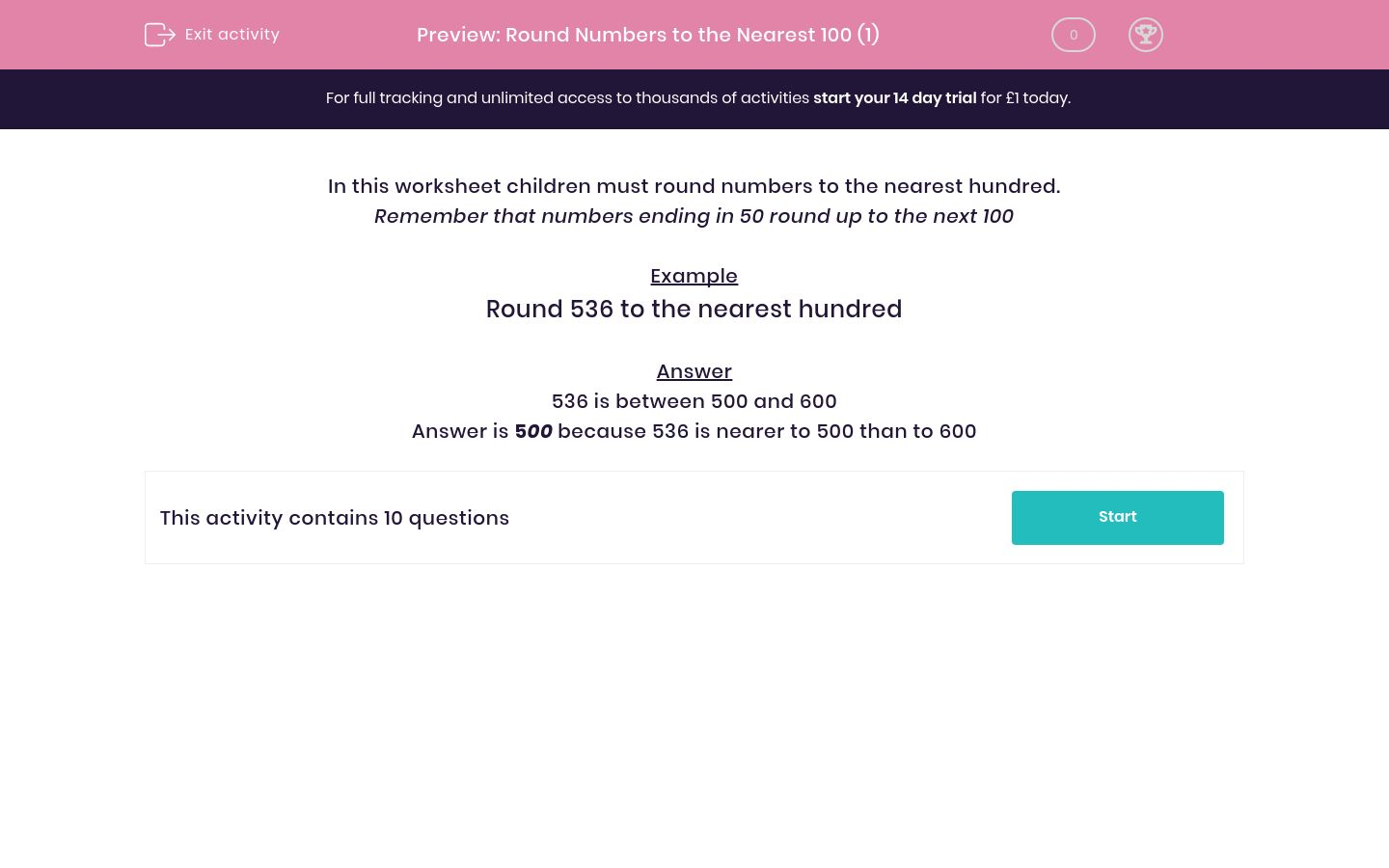# Round Numbers to the Nearest 100 (1)

In this worksheet, students round numbers to the nearest hundred.Key stage:  KS 2

Curriculum topic:   Maths and Numerical Reasoning

Curriculum subtopic:   Place Value

Difficulty level:### QUESTION 1 of 10

In this worksheet children must round numbers to the nearest hundred.

Remember that numbers ending in 50 round up to the next 100

Example

Round 536 to the nearest hundred

536 is between 500 and 600

Answer is 500 because 536 is nearer to 500 than to 600

Round the number to the nearest hundred

384 rounded to the nearest hundred is __

Round the number to the nearest hundred

870 rounded to the nearest hundred is __

Round the number to the nearest hundred

669 rounded to the nearest hundred is __

Round the number to the nearest hundred

268 rounded to the nearest hundred is __

Round the number to the nearest hundred

416 rounded to the nearest hundred is __

Round the number to the nearest hundred

443 rounded to the nearest hundred is __

Round the number to the nearest hundred

550 rounded to the nearest hundred is __

Round the number to the nearest hundred

552 rounded to the nearest hundred is __

Round the number to the nearest hundred

750 rounded to the nearest hundred is __

Round the number to the nearest hundred

748 rounded to the nearest hundred is __

• Question 1

Round the number to the nearest hundred

384 rounded to the nearest hundred is __

400
• Question 2

Round the number to the nearest hundred

870 rounded to the nearest hundred is __

900
• Question 3

Round the number to the nearest hundred

669 rounded to the nearest hundred is __

700
• Question 4

Round the number to the nearest hundred

268 rounded to the nearest hundred is __

300
• Question 5

Round the number to the nearest hundred

416 rounded to the nearest hundred is __

400
• Question 6

Round the number to the nearest hundred

443 rounded to the nearest hundred is __

400
• Question 7

Round the number to the nearest hundred

550 rounded to the nearest hundred is __

600
• Question 8

Round the number to the nearest hundred

552 rounded to the nearest hundred is __

600
• Question 9

Round the number to the nearest hundred

750 rounded to the nearest hundred is __

800
• Question 10

Round the number to the nearest hundred

748 rounded to the nearest hundred is __

700
---- OR ----

Sign up for a £1 trial so you can track and measure your child's progress on this activity.

### What is EdPlace?

We're your National Curriculum aligned online education content provider helping each child succeed in English, maths and science from year 1 to GCSE. With an EdPlace account you’ll be able to track and measure progress, helping each child achieve their best. We build confidence and attainment by personalising each child’s learning at a level that suits them.

Get started